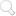# Shading Coefficients

The total shading coefficient is a measure of the total amount of heat passing through the glazing (known as the total solar heat transmittance) compared with that through a single clear glass. The shading coefficient (SC) is derived by comparing the solar radiant heat transmission properties of any glass with a clear float glass having a total solar heat transmittance of 0.87 (i.e. clear float glass about 4mm thick).

The shading coefficient (SC) can be separated into long-wave and short-wave components, whose values are derived in exactly the same way, by comparing with the same property of 4mm clear float:
• The short wavelength shading coefficient (SWSC) is the direct solar heat transmittance divided by 0.87.
• The long wavelength shading coefficient (LWSC) is the fraction of the absorptance released inwards, again divided by 0.87.